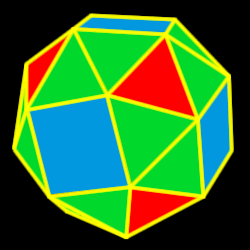# Snub Cuboctahedron

Properties of the snub cuboctahedron: Number of faces, edges and dihedral angle measure

The snub cuboctahedron is created by either truncating (cutting off) tips
of the cube or the octahedron.38 total faces:
32 equilateral triangles and 6 squares

24 vertices:
4 triangles and 1 square

60 edges

Dihedral angles:
142 degrees, 59 minutes for the sqr-tri angle and 153 degrees, 14 minutes for the tri-tri angle